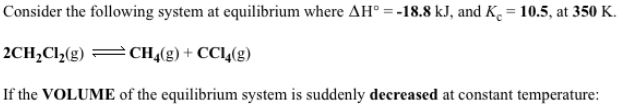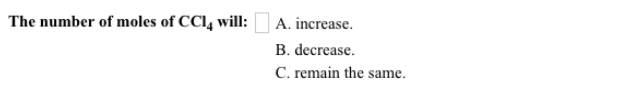# Consider the following system at equilibrium where ΔH° = -18.8 kJ, and Kc = 10.5 at 350 K. 2CH2Cl2(g) ⇌ CH4(g) + CCl4(g) If the VOLUME of the equilibrium system suddenly decreased at constant temperature: The number of moles of CCl4 will: a) increase b) decrease c) remain the same